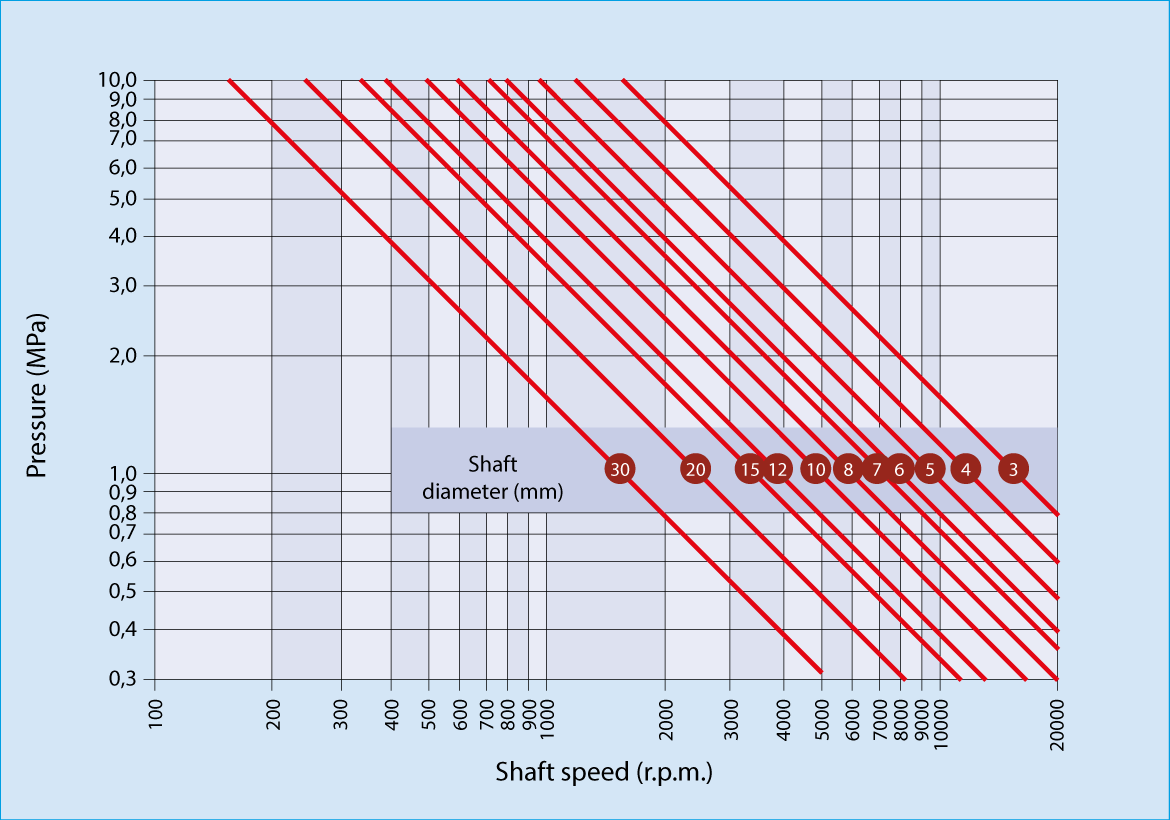# Calculations for SELFOIL® bearings

The suitability of SELFOIL® bearings for a given system can be verified by checking the values of pressure and shaft speed in this chart, as shown in the examples below.Example 1

Data:A shaft/bearing system works at a shaft speed of 800 r.p.m. What is the maximum permissible load for a SELFOIL® bearing of inner diameter 20 mm and length 25 mm?

Solution: The shaft diameter is considered the same as the bearing inner diameter.
The speed of 800 r.p.m. and the shaft diameter of 20 mm are both placed in the load/speed chart. A maximum workin pressure of 3,0 MPa is obtained.Then, the projected surface is calculated as S — L — 20 mm • 25 mm — 500 mrn&sup2 . Finally, as pressure is P z F/S, by solving the equation, the load is obtained as F P S 3,0 MPa • 500 mrry z 1.500 N. So the maximum admissible load will be 1.500 N (150 Kg aproximately).

Example 2

Calculation of the minimum length of a SELFOlL® bearing

Data: A shaft/bearing system has a shaft of diameter 20 mm that rotates at a speed of 600 r.p.m. speed under a load of 1,500 N. What is the minimum length that a SELFOIL® bearing should have in order to work properly under these conditions?

Solution: The speed of 600 r.p.m. and the shaft diameter of 20 mm are both placed in the load/speed chart. A maximum working pressure of 4.0 MPa is obtained. As pressure is P = F/S, the projected surface is obtained as S = F/P = 1,500 N / 4.0 MPa = 375 mm&sup2. The shaft diameter is the taken as being equal to the bearing inner diameter. Since the projected surface S = Øint· L, the bearing length is calculated as L = S/Øint = 375 mm&sup2 / 20 mm = 18.75 mm. So the minimum bearing length will be 18.75 mm.